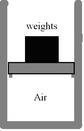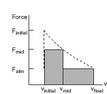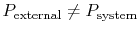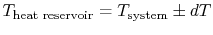Thermodynamics and Propulsion

4.3 Features of reversible processes

[VW, S & B: 6.3-6.4]

Reversible processes are idealizations or models of real processes. One familiar and widely used example is Bernoulli's equation, which you saw in Unified. They are extremely useful for defining limits to system or device behavior, for enabling identification of areas in which inefficiencies occur, and in giving targets for design.

An important feature of a reversible process is that, depending on the process, it represents the maximum work that can be extracted in going from one state to another, or the minimum work that is needed to create the state change.

Let us consider processes that do work, so that we can show that the reversible one produces the maximum work of all possible processes between two states. For example, suppose we have a thermally insulated cylinder that holds an ideal gas, Figure 4.9. The gas is contained by a thermally insulated massless piston with a stack of many small weights on top of it. Initially the system is in mechanical and thermal equilibrium.Consider the following three processes, shown in Figure 4.10:

1. All of the weights are removed from the piston instantaneously and the gas expands until its volume is increased by a factor of four (a free expansion).
2. Half of the weight is removed from the piston instantaneously, the system is allowed to double in volume, and then the remaining half of the weight is instantaneously removed from the piston and the gas is allowed to expand until its volume is again doubled.
3. Each small weight is removed from the piston one at a time, so that the pressure inside the cylinder is always in equilibrium with the weight on top of the piston. When the last weight is removed, the volume has increased by a factor of four.Maximum work (proportional to the area under these curves) is obtained for the quasi-static expansion.

To reiterate:

• The work done by a system during a reversible process is the maximum work we can get.
• The work done on a system in a reversible process is the minimum work we need to do to achieve that state change.

A process must be quasi-static (quasi-equilibrium) to be reversible. This means that the following effects must be absent or negligible:

1. Friction: Ifwe would have to do net work to bring the system from one volume to another and return it to the initial condition (recall Section 1.3.3.)
2. Free (unrestrained) expansion.
3. Heat transfer through a finite temperature difference.Suppose we have heat transfer from a high temperature to a lower temperature as shown in Figure 4.11. How do we restore the situation to the initial conditions? One thought would be to run a Carnot refrigerator to get an amount of heat,, from the lower temperature reservoir to the higher temperature reservoir. We could do this but the surroundings, again us, would need to provide some amount of work (which we could find using our analysis of the Carnot refrigerator). The net (and only) result at the end of the combined process would be a conversion of an amount of work into heat. For reversible heat transfer from a heat reservoir to a system, the temperatures of the system and the reservoir must be. In other words the difference between the temperatures of the two entities involved in the heat transfer process can only differ by an infinitesimal amount,.

While all natural processes are irreversible to some extent, it cannot be emphasized too strongly that there are a number of engineering situations where the effect of irreversibility can be neglected and the reversible process furnishes an excellent approximation to reality.

The second law, which is the next topic we address, allows us to make a quantitative statement concerning the irreversibility of a given physical process.Muddy Points

Is heat transfer across a finite temperature difference only irreversible if no device is present between the two to harvest the potential difference? (MP 4.5)

Douglas Quattrochi 2006-08-06